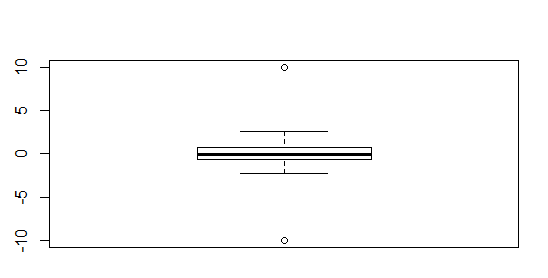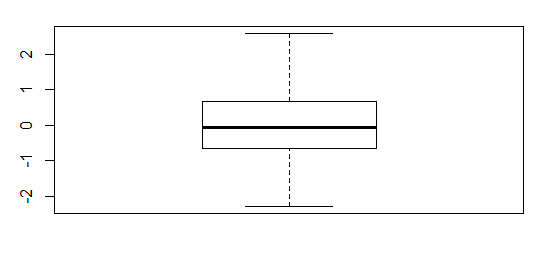2 views

I've got some multivariate data of beauty vs ages. The ages range from 20-40 at intervals of 2 (20, 22, 24....40), and for each record of data, they are given age and a beauty rating from 1-5. When I do boxplots of this data (ages across the X-axis, beauty ratings across the Y-axis), there are some outliers plotted outside the whiskers of each box.

I want to remove these outliers from the data frame itself, but I'm not sure how R calculates outliers for its box plots. Below is an example of what my data might look like.by

To remove outliers from a dataset(generally not preferred), you can use the following function:

df[!df %in% boxplot.stats(df)\$out]

For example:

Boxplot with outliers:

set.seed(100)

x <- rnorm(100)

x <- c(-10, x, 10)

boxplot(x)Boxplot without outliers:
y <- x[!x %in% boxplot.stats(x)\$out]
boxplot(y)